Next: Possible mechanism Up: Annotation Previous: Conclusions to Chapter 4   Contents

# On possible frequency parametrization

In Appendixes some particular hypotheses will be considered. Practically, they do not connected with the criticism of relativity theory from the main part of this book; they only demonstrate nonuniqueness of the SRT approach and a possibility of the frequency parametrization of all formulas. This is the only claim of these appendixes in the book, since we will use incorrect SRT methods (their error was proved in the main part of the book). The author attempted to discuss ideas from Appendixes A and B (plus a part of analysis of the Michelson experiment from Chapter 3) in several well-known journals in 1993-1999. The result was the same: either the work did diplomatically not be considered right away or the approximate answer was "nobody discovered these things in relativity theory and quantum electrodynamics, but the exactness of predictions of these theories was huge". How can theorist discover anything new (instead of explanation its "by late mind")? He must assume some fact and test corollaries from his assumption. But nobody attempts to assume the possibility of frequency dependence of light speed. Besides, the case in point was the precision on one-two orders large than the existing modern precision of experiments. Such a precision can be reached in the immediate future; though there exist experiments, which need in precision on some dozens of orders large than existing one, but they have been seriously discussing in physics. The author was tired to waste the time at last, and a decision was taken to test the "huge precision of relativity theory" (at the same time remembering a student dissatisfaction by this theory). As a result, my first critical article appeared (and now this book too). So, plus and minus together are presented everywhere.

Now we proceed to discussion of a possible frequency dependence of light speed. It is well known that when particles are in vacuum, there occur various processes, such as the appearance of virtual pairs (a particle and its antiparticle); many interaction processes are described in terms of such virtual pairs. Also, light influences vacuum properties during its propagation (in particular, vacuum polarization takes place). Therefore, by the reciprocity principle there must be a reverse action of vacuum polarization on the light propagation. As a consequence, the light (at a certain frequency) is bound to travel through the vacuum as "the medium" with some certain permittivity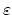, which is determined by this light itself; that is,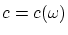.

The generalization of the Maxwell equations by adding the mass term explicitly to the Maxwell Lagrangian is known to lead to the Proca equations in the Minkowski space (in the modern view). An electromagnetic wave propagating through the medium is influenced by the latter and this effect is manifested via the generation of massive photons . Even with constant phase speed assumed, an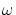-dependence of the group speed (dispersion in vacuum) is known to arise: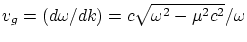, whereis the rest mass of the photon. However, the question of mass generation and the gauge theories will not be discussed in these Appendixes. Our aim is just to represent some physical reflections about light velocity and attendant questions.

The questions arise here: 1) How can the-dependence be evaluated or measured? 2) Why has it not yet been found, and 3) What corollaries follow?

There exist various methods for measuring light speed: astronomical methods, the method of interruptions, the rotating mirror method, the radio geodetic method, the method of standing waves (the resonator), the independent measurements of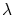and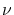, and so on. At the present time, the last method [59,67] is the most precise; it is used by the Bureau of Standards for measuring light speed to eight significant digits. However, an important problem arises in this approach . Besides, it must be emphasized that this method is principally limited: either it can be connected with local (inside a device) speed of light only, or it can bear no relationship to light speed at all in the case if light by itself does not represent a pure wave. Why other methods are inadequate (fail to detect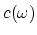dependence) is clear from the previous Chapters and will be clear from given Appendixes for one particular hypothesis.

In further consideration we will follow SRT methods (we will forget their error for the time being, but present an "apparent effect" for two systems under an additional condition only - under the condition of the choice of the Einstein synchronization method). Recall that in deriving the corollaries of SRT (transformation laws, for example) the notion of the interval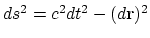is used. Here it is necessary to make two methodological remarks. First, even the equality of intervalsis nothing more than one possible hypotheses, since only a single point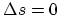remains trustworthy (if we suppose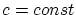). For example, we could pick any natural number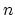and equate the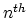degrees,, and obtain different "physical laws". Or, we could consider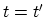, but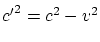, i.e.(the apparent velocity of mutual motion is different for different observers). Such a choice results in coinciding of the relativistic longitudinal Doppler effect with the classical expression. Similar exotic systems could be as much intrinsically self-consistent as the SRT (i.e. for two marked objects only!), and only the experiments could demonstrate which choices are nothing more than theoretical fabrications. We shall not discuss all such exotic hypotheses here.

Second, in the usage of interval, the following point is not emphasized: the specific light, propagating from one point to another, is used in this case, i.e. the valueshould be substituted in the expression for the interval. But in such a case, assuming proportionality of intervals from textbooks, an indeterminate relation is obtained:and even the equality of intervals cannot be proved. This indeterminate relation is associated with the still "unknown" Doppler law, so there is again need for reference to experiments. Thus, theoretical constructions proceeding from intrinsic individual principles are not unique. Since generally accepted derivations results in some corollaries that are confirmed experimentally (for particle dynamics within some precision, for example), we shall rely upon this method, but modify it with regard to the possibledependence.

Physically, this approach implies the following: The apparent result of some measurement depends on the measurement technique; and the calculated result depends,in particular, on the synchronization procedure for timepieces in different frames. According to an idea from this Appendix, no "unique interaction propagation speed" exists (but). If light of some definite frequencyis used for Einstein synchronization of timepieces in the different frames, the result of any experiment depends on. For example, if some process with characteristic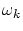takes place in a system, then it is natural to watch the system by using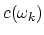(just as the signal propagates). If two systems moving relative to each other are studied in the experiment, then two quantitiesand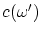(for each frame) appear in formulae. This is due to the fact, that the same light possesses different frequencies in systems moving relative to each other. As this takes place, the quantitiesand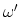are related to each other by the Doppler effect (see below). It is interesting to note the following circumstance. If several various processes with characteristic frequencies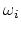take place in the system, then the observers moving with respect to each other will see (at the same point) various pictures of events (the apparent effect). In the subsequent theoretical description we shall follow [4,17].

Letbe the frequency of signal propagation in some system. Substituting(instead of) into the four-dimensional interval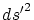for the intrinsic system andinto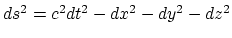for the system of observation, it follows fromthat the intrinsic time (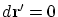) can be found from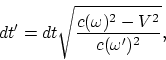(A.1)

but the formula for the intrinsic length retains its validity. We note again, that it is "a visible effect" only. In an arbitrary mathematical expression coefficients can be transfered (according to some rules) from the left-hand side in the right-hand side of the expression and vice versa (all these expressions are equivalent). Then, how can it be determined: accelerats time at one observer or, contrary, decelerats at other one (and increased or decreased lengths)? Simply, if somebody were said to you that just yours time is decelerated by different manner relative several objects, you would right away understand senselessness of the infinite number of such "informations". However relativists say that yours time is OK, but simply "somebody has something somewhere far off", and many people calm right away and continue to listen "the fairy-tales".

To derive the Lorentz transformations, one can use rotation in theplane: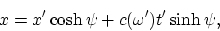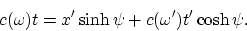Using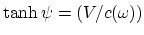, it follows that the Lorentz transformation reduces to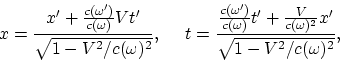(A.2)

where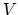is the system velocity. Writing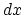and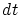in the expression (A.2) and finding, one obtains, that the transformations for velocity change into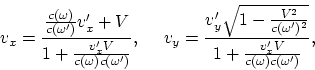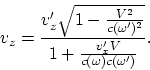(A.3)

It follows that for the motion along the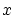axis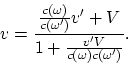(A.4)

We see that the maximum of velocity is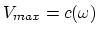, whereis the light frequency in the intrinsic system. Note that all formulae lead to the correct composition law for motion along the same straight line (the transformation from frame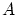toand fromto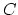yields the same result as the transformation fromto). Recall that, in accord with considerations given in the main part of the book, quantities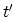and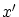in formulas (A.1), (A.2) have no intrinsic physical meaning (they are fictitious auxiliary quantities). Formula (A.4), by analogy with formula (1.5), can be re-written as(A.5)

This form most clearly reveals the essence of this expression (the apparent effect). The formula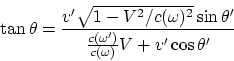(A.6)

describes the change of the velocity direction. The relativistic expression for the light aberration holds (the substitution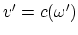). To be on the safe side, we are reminded that the relativistic expression for the stellar aberration is approximate. The transformations of 4-vectors are also valid. From here follow the transformations of the wave four-vector: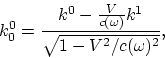As a result, the Doppler effect can be obtained from(A.7)

Note, that from here follows the dependence of light speed on the system motion (different frequenciescorrespond to different systems). However, as we shall see in the next Appendix, this effect is negligible for the visible region. Relativists declare that the expression of the Doppler effect contains the relative velocity. It is false. Let an explosion occur at some point on the Earth, and let some line of emission be radiated in short time. Let a receiver at the Pluton catch the signal. At which a moment must we determine this mythical relative velocity? The receiver can not see in the direction to the Earth at the moment of explosion, and the source not exists at the moment of the signal receiving, and the Earth will be turned to the back side. Even in the absence of medium, we obtain, instead of the relative velocity, the difference of absolute velocities at the moment of emission and at the moment of signal receiving (and it is not the same!). But the real result can be obtained in the real experiment only.

The energy-momentum vector transforms as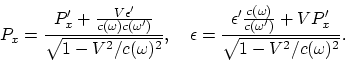(A.8)

There should be a closer analogy between light propagation through vacuum and through a medium.

1)  Various packets of waves diffuse in vacuum variously.

2)  Light dispersion in vacuum imposes a fundamental limitation on the degree of ray parallelism.

3)  There is light dissipation in vacuum; that is, the intensity of light decreases as it propagates in vacuum.

4)  Light "ages"; that is, the frequency of light decreases as it propagates in vacuum. This phenomenon bears on the paradox (Olbers) "why does the sky not flame?" and contributes to the red shift; that is, a correction of the world evolution concept is in order. Since we are factually dealing with an alternative explanation of the red shift, this effect appears to be very small, and, at present, it cannot be confirmed in laboratoty experiments: the red shift of lines for cosmic objects is already detected by the most precise optical methods and it becomes to be noticeable for very distant objects only, such the ones that distances to theirs cannot be found even on the Earth's orbit base (on the triangle). Recall in this connection that even an order of the value of Habble constant had already been corrected.

Passing to quantum electrodynamics, the substitution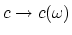needs to be done in all derivations. For example, this dependence appears in the uncertainty relationin the condition for classical descriptionand in numerous formulae.

If some formula describes the-dependence, then it can substantially change. As an example, we consider the emission and absorption of photons. The new coefficient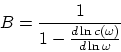appears in the expression for the number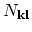of photons with a given polarization: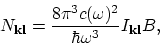and in the relation for probabilities (of absorption, induced and spontaneous emission)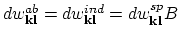. Quantityappears in Einstein's coefficients.

Using the substitution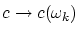for natural field oscillations, one obtains the expression for the Fourier component of the photon propagator: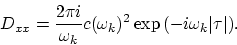We cannot findwithout knowledge of the explicit dependence. The explicit form of the-dependence is necessary to find a net result for various cross-sections (for scattering, for the origin of a pair, for disintegration, etc.). As a first approximation, the substitutioncan be made in the well-known formulae.

There we shall discuss the possible-dependence.Next: Possible mechanism Up: Annotation Previous: Conclusions to Chapter 4   Contents
Sergey N. Arteha